Subsections

# 9.3 The R language

R is a popular programming language for working with and analyzing data.

As with the other computer languages that we have dealt with in this book, we have two main topics to cover for R: we need to learn the correct syntax for R code, so that we can write code that will run; and we need to learn the semantics of R code, so that we can make the computer do what we want it to do.

R is a programming language, which means that we can achieve a much greater variety of results with R compared to the other languages that we have seen. The cost of this flexibility is that the syntax for R is more complex and, because there are many more things that we can do, the R vocabulary that we have to learn is much larger.

In this section, we will look at the basics of R syntax--how to write correct R code. Each subsequent section will tackle the meaning of R code by focusing on a specific category of tasks and how they are performed using R.

## 9.3.1 Expressions

R code consists of one or more expressions.

An expression is an instruction to perform a particular task. For example, the following expression instructs R to add the first four odd numbers together.

 > 1 + 3 + 5 + 7   16 

If there are several expressions, they are run, one at a time, in the order they appear.

The next few sections describe the basic types of R expressions.

## 9.3.2 Constant values

The simplest sort of R expression is just a constant value, typically a numeric value (a number) or a character value (a piece of text). For example, if we need to specify a number of seconds corresponding to 10 minutes, we specify a number.

 > 600   600 

If we need to specify the name of a file that we want to read data from, we specify the name as a character value. Character values must be surrounded by either double-quotes or single-quotes.

 > "http://www.census.gov/ipc/www/popclockworld.html"   "http://www.census.gov/ipc/www/popclockworld.html" 

As shown above, the result of a constant expression is just the corresponding value and the result of an expression is usually printed out on the screen.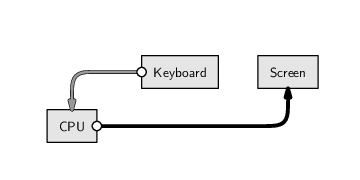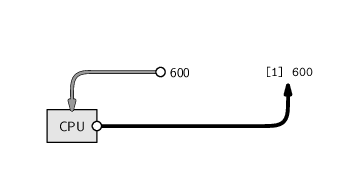## 9.3.3 Arithmetic

An example of a slightly more complex expression is an arithmetic expression for calculating with numbers. R has the standard arithmetic operators:

 + addition. - subtraction. * multiplication. / division. ^ exponentiation.

For example, the following code shows the arithmetic calculation that was performed in Section 9.1 to obtain the rate of growth of the world's population--the change in population divided by the elapsed time. Note the use of parentheses to control the order of evaluation. R obeys the normal BODMAS rules of precedence for arithmetic operators, but parentheses are a useful way of avoiding any ambiguity, especially for a human audience.

 > (6617747987 - 6617746521) / 10   146.6 

## 9.3.4 Conditions

A condition is an expression that has a yes/no answer--for example, whether one data value is greater than, less than, or equal to another. The result of a condition is a logical value: either TRUE or FALSE.

R has the standard operators for comparing values, plus operators for combining conditions:

 == equality. > and >= greater than (or equal to). < and <= less than (or equal to). != inequality. && logical and. || logical or. ! logical not.

For example, the following code asks whether the second population estimate is larger than the first.

 > pop2 > pop   TRUE 

The code below asks whether the second population estimate is larger than the first and the first population estimate is greater than 6 billion.

 > (pop2 > pop) && (pop > 6000000000)   TRUE 

The parentheses in this code are not necessary, but they make the code easier to read.

## 9.3.5 Function calls

The most common and most useful type of R expression is a function call. Function calls are very important because they are how we use R to perform any non-trivial task.

A function call is essentially a complex instruction, and there are thousands of different R functions that perform different tasks. This section just looks at the basic structure of a function call; we will meet some important specific functions for data manipulation in later sections.

A function call consists of the function name followed by, within parentheses and separated from each other by commas, expressions called arguments that provide necessary information for the function to perform its task.

The following code gives an example of a function call that makes R pause for 10 minutes (600 seconds).

 > Sys.sleep(600) 

The various components of this function call are shown below:

 function name: Sys.sleep(600) parentheses: Sys.sleep (600 ) argument: Sys.sleep( 600)

The name of the function in this example is Sys.sleep (this function makes the computer wait, or “sleep”, for a number of seconds). There is one argument to the function, the number of of seconds to wait, and in this case the value supplied for this argument is 600.

Because function calls are so common and important, it is worth looking at a few more examples to show some of the variations in their format.

The writeLines() function saves text values into an external file. This function has two arguments: the text to save and the name of the file that the text will be saved into. The following expression shows a call to writeLines() that writes the text "146.6" into a file called popRate.txt.

 > writeLines("146.6", "popRate.txt") 

The components of this function call are shown below:

 function name: writeLines("146.6", "popRate.txt") parentheses: writeLines ("146.6", "popRate.txt" ) comma between arguments: writeLines("146.6" , "popRate.txt") first argument: writeLines( "146.6", "popRate.txt") second argument: writeLines("146.6", "popRate.txt")

This example demonstrates that commas must be placed between arguments in a function call. The first argument is the text to save, "146.6", and the second argument is the name of the text file, "popRate.txt".

The next example is very similar to the previous function call (the result is identical), but it demonstrates the fact that every argument has a name and these names can be specified as part of the function call.

 > writeLines(text="146.6", con="popRate.txt") 

The important new components of this function call are shown below:

 first arg. name: writeLines( text="146.6", con="popRate.txt") first arg. value: writeLines(text= "146.6", con="popRate.txt") second arg. name: writeLines(text="146.6", con="popRate.txt") second arg. value: writeLines(text="146.6", con= "popRate.txt")

When arguments are named in a function call, they may be given in any order, so the previous function call would also work like this:

 > writeLines(con="popRate.txt", text="146.6") 

The final example in this section is another variation on the function call to writeLines().

 > writeLines("146.6")  146.6 

The point of this example is that we can call the writeLines() function with only one argument. This demonstrates that some arguments have a default value, and if no value is supplied for the argument in the function call, then the default value is used. In this case, the default value of the con argument is a special value that means that the text is displayed on the screen rather than being saved to a file.

There are many other examples of function calls in the code examples throughout the remainder of this chapter.

## 9.3.6 Symbols and assignment

Anything that we type that starts with a letter, and which is not one of the special R keywords, is interpreted by R as a symbol (or name).

A symbol is a label for an object that is currently stored in RAM. When R encounters a symbol, it extracts from memory the value that has been stored with that label.

R automatically loads some predefined values into memory, with associated symbols. One example is the predefined symbol pi, which is a label for the the mathematical constant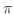.

 > pi   3.141593 

The result of any expression can be assigned to a symbol, which means that the result is stored in RAM, with the symbol as its label.

For example, the following code performs an arithmetic calculation and stores the result in memory under the name rateEstimate.

 > rateEstimate <- (6617747987 - 6617746521) / 10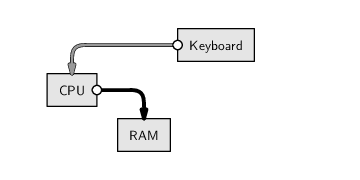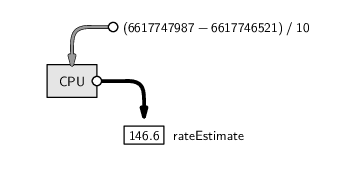The combination of a less-than sign followed by a dash, <-, is called the assignment operator. We say that the symbol rateEstimate is assigned the value of the arithmetic expression.

Notice that R does not display any result after an assignment.

When we refer to the symbol rateEstimate, R will retrieve the corresponding value from RAM. An expression just consisting of a symbol will result in the value that is stored with that name being printed on the screen.

 > rateEstimate   146.6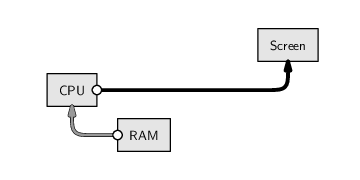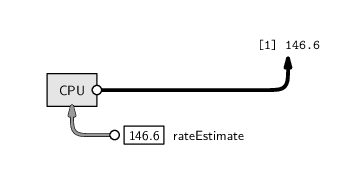In many of the code examples throughout this chapter, we will follow this pattern: in one step, we will calculate a value and assign it to a symbol, which produces no display; then, in a second step, we will type the name of the symbol on its own to display the calculated value.

We can also use symbols as arguments to a function call, as in the following expression, which converts the numeric rate value into a text value.

 > as.character(rateEstimate)   "146.6" 

The value that has been assigned to rateEstimate, 146.6, is retrieved from RAM and passed to the as.character() function, which produces a text version of the number.

In this case, we do not assign the result of our calculation, so it is automatically displayed on the screen.

For non-trivial tasks, assigning values to symbols is vital because we will need to perform several steps in order to achieve the ultimate goal. Assignments allow us to save intermediate steps along the way.

## 9.3.7 Keywords

Some symbols are used to represent special values. These predefined symbols cannot be re-assigned.

NA

This symbol represents a missing or unknown value.
Inf

This symbol is used to represent infinity (as the result of an arithmetic expression).

 > 1/0   Inf 

NaN

This symbol is used to represent an arithmetic result that is undefined (Not A Number).

 > 0/0   NaN 

NULL

This symbol is used to represent an empty result. Some R functions do not produce a result, so they return NULL.
TRUE and FALSE

These symbols represent the logical values “true” and “false”. The result of a condition is a logical value.

 > pop2 > pop   TRUE 

## 9.3.8 Flashback: Writing for an audience

Chapter 2 introduced general principles for writing computer code. In this section, we will look at some specific issues related to writing scripts in R.

The same principles, such as commenting code and laying out code so that it is easy for a human audience to read, still apply. In R, a comment is anything on a line after the special hash character, #. For example, the comment in the following line of code is useful as a reminder of why the number 600 has been chosen.

Sys.sleep(600) # Wait 10 minutes


Indenting is also very important. We need to consider indenting whenever an expression is too long and has to be broken across several lines of code. The example below shows a standard approach that left-aligns all arguments to a function call.

popText <- gsub('^.*<div id="worldnumber">|</div>.*\$',
"", popLine)


It is also important to make use of whitespace. Examples in the code above include the use of spaces around the assignment operator (<-), around arithmetic operators, and between arguments (after the comma) in function calls.

## 9.3.9 Naming variables

When writing R code, because we are constantly assigning intermediate values to symbols, we are forced to come up with lots of different symbol names. It is important that we choose sensible symbol names for several reasons:

1. Good symbol names are a form of documentation in themselves. A name like dateOfBirth tells the reader a lot more about what value has been assigned to the symbol than a name like d, or dob, or even date.
2. Short or convenient symbol names, such as x, or xx, or xxx should be avoided because it too easy to create errors by reusing the same name for two different purposes.

Anyone with children will know how difficult it can be to come up with even one good name, let alone a constant supply. However, unlike children, symbols usually have a specific purpose, so the symbol name naturally arises from a description of that purpose. A good symbol name should fully and accurately represent the information that has been assigned to that symbol.

One problem that arises is that a good symbol name is often a combination of two or more words. One approach to making such symbols readable is to use a mixture of lowercase and uppercase letters when typing the name; treat the symbol name like a sentence and start each new word with a capital letter (e.g., dateOfBirth). This naming mechanism is called “camelCase” (the uppercase letters form humps like the back of a camel).

## Recap

A programming language is very flexible and powerful because it allows us to control the computer hardware as well as computer software.

R is a programming language with good facilities for working with data.

An instruction in the R language is called an expression.

Important types of expressions are: constant values, arithmetic expressions, function calls, and assignments.

Constant values are just numbers and pieces of text.

Arithmetic expressions are typed mostly as they would be written, except for division and exponentiation operators.

Function calls are instructions to perform a specific task and are of the form:

functionName(argument1, argument1)

An assignment saves a value in computer memory with an associated label, called a symbol, so that it can be retrieved again later. An assignment is of the form:

symbol <- expression

R code should be written in a disciplined fashion just like any other computer code.

Paul Murrell